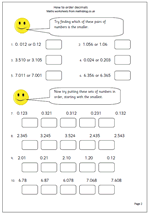## Resource of the Week: short division of decimals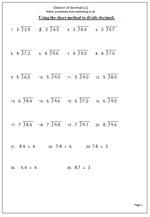This week I would like to look at a page of practice on using the short method of division of decimals. The numbers being divided are just units and tenths which helps with getting the method correct.

There are arguments for and against putting the decimal point in before you start, or leaving it until you have reached that point in the question. it does not matter as long as it is inserted correctly.

One of the best ways to be fluent with this method is to talk it through out loud. If we look at question 2 which is 4.5 divided by 3, the verbal stages are:

a. How many 3s in 4?

b. 1 times 3 is 3 so there is 1 with a remainder of 1.

c. Place the 1 on the answer line, immediately above the 3.

d. Place the decimal point just above the answer line so it can be clearly seen.

e. The remainder 1 is placed just in front of the 5 (usually written smaller).

f. How many 3s in 15?

g. 3 x 5 is 15 so the answer is 5.

h. Place the 5 on the answer line, immediately above the 5 (tenths).

Division of decimals (1)

## Year 4 maths worksheet on ordering decimal fractions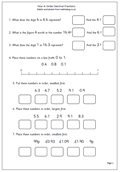One of the most important concepts in maths is to understand that the value of a digit depends on its place in the number – otherwise we could only possibly have 9 numbers and zero! So the digit 2 in 120 has a different value than the 2 in 210. The 2 in 120 has a value of 20, whilst the 2 in 210 has a value of 200.

By Year 4 children should be applying this idea to decimals. The first column to the right of the decimal point is tenths so the 1 in 2.1 has a value of one tenth and the 1 in 2.01 has a value of one hundredth. This is best shown with money: one penny is £0.01, or one hundredth, whilst 10p is £0.10 or one tenth of a pound. (It is also important that we always put two digits after the decimal when using money, but not necessarilyat other times.)

This maths worksheets looks at both place value and ordering simple decimal fractions, using the concepts above.

year 4 worksheet: Order decimal fractions

## More help with ordering decimals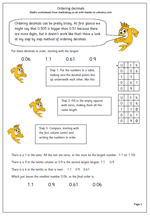I have published worksheets on ordering decimals before, but this page, lent to me from urbrainy.com is a very neat way of making sure that decimals are put in order correctly.

It suggests a very simple, step by step, approach which includes:

1. putting the numbers underneath each other, in a table, making sure that the decimals all line up.

2. filling any empty spaces with zeros, making them all the same length, with the same number of digits.

3. beginning to compare, starting with the units column.

Children who have not used decimals may well think that the size of a number depends on the number of digits so comparing decimals can be tricky, as a three digit number such as 0.19 is smaller than a 2-digit number such as 0.2.

Order decimals

## Year 4 decimals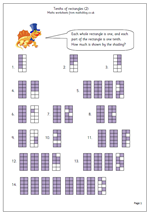One of the best ways to introduce decimals is by working with money which shows the idea of tenths (10p) and hundredths (1p). Another good way is to use shading of shapes and this is the second page which does this. A series of rectangles is shown, each divided into ten equal parts: each part being one tenth. This is a very clear illustration of what tenths are and children should find it relatively easy to write down how much is shown: this could also be used on a whiteboard as a whole class activity at the start of the lesson.

It could also be used to show that 1/10 is the same as 0.1, 2/10 the same as 0.2 etc.

This is the second page of Tenths of Rectangles and can be found in our year 4 Counting and Numbers category.

Tenths of rectangles (2)

## Year 4 maths worksheet: Tenths of rectangles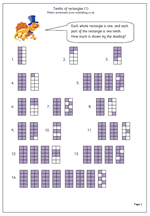A good way of working with decimals is to use shapes which are divided into ten equal parts. The shape is one whole unit and each part is one tenth, which can be written as 0.1.

This page has a selection of shapes and asks the child to write down the amount that has been shaded. Where all parts of a shape have been shaded this counts as ten tenths, or one whole one.

Whilst printed pages nearly always show the decimal point situated on the line most schools show it as half way up the line: the most important thing is that it shows up clearly.

This page can be found in our Year 4 maths worksheets in the Counting and Number category.

Tenths of rectangles (1)

## Multiply a decimal by a single digit (2)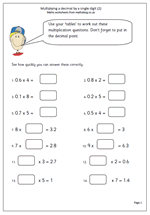Here we have two more pages of practice at multiplying a decimal by a single digit. The first six questions on each page are straightforward enough but the later questions may prove to be trickier.

For example: 8 x ? = 3.2 could appear to be quite difficult to work out. Because 3.2 is a smaller than 8 the missing number must be less than 1. if tables are known then 8 x 4 = 32 is known and it should be easy to then work out that 8 x 0. 4 = 3.2.

These two worksheets can be found in our Year 6 Knowing Number facts section, along with more pages on multiplying, doubling and halving.

More_multiplying_decimals  (2)

More_multiplying_decimals  (3)

## Multiplying a decimal by a single digit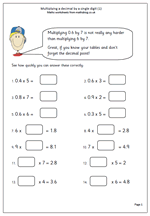Some quick mental arithmetic work here, ideal for homework. Multiplying 0.6 by 7 is not really any harder than multiplying 6 by 7, as long as you have a good understanding of place value.

Watch out for a common error with these, an example of which is:

0.8 x 7 = 0.56

In this case, whilst the times table is known what to do about the decimal point remains a mystery. if you come across this error it would be a very good idea to go back to basics and look at multiplying and dividing by 10 across the decimal point.

This is a good page for year 6 revision but could be used by younger children who have a sound understanding of decimals. It can be found in our year 6 Knowing number facts section.

More_multiplying_decimals (1)

## Year 6 maths worksheet: Calculator patterns and recurring decimals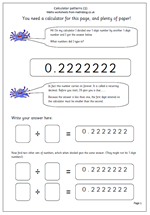The calculator can prove to be a great tool for those who are fascinated by numbers and these pages give us just one example of this.

It uses simple division sums to produce interesting recurring decimals. For example:

1/9 is 0.111111

2/9 is 0.22222

3/9 is 0.33333

and so on.

It also asks children to use their knowledge of equivalent fractions to see if they can come up with other division questions which also create these patterns. As 1/9 is equivalent to 2/18 then this fraction will also create the same pattern.

A further extension is to ask why this happens and this can be answered by doing the division by written methods where it will soon be noticed that the same remainder keeps recurring, hence the recurring or never ending answer. The numbers can also be continued: 10/9 = 1.111111 etc. Fascinating!!

(A philosophical extra to ask  is why doesn’t 9/9 give 0.999999?)

These pages can be found in our Using and Understanding Maths section for Year 6.

Recurring decimals (1)

## Year 6 worksheet: Add decimals mentally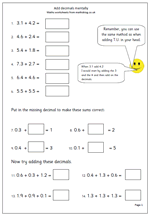A straightforward mental arithmetic page on adding decimals. The process is very much the same as adding two 2-digit whole numbers. When adding in our heads we usually start with the largest numbers, which, of course, is the opposite of the way we do it on paper. This needs pointing out to children as many, even in Year 6, lack the strategies necessary for mental addition.

When adding 3 decimals there are several different strategies that can be used, including:

looking for pairs that make 1

starting with the largest number

counting on

knowing doubles etc.